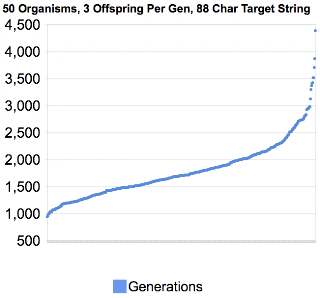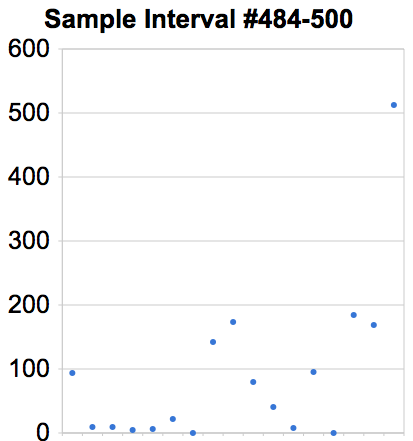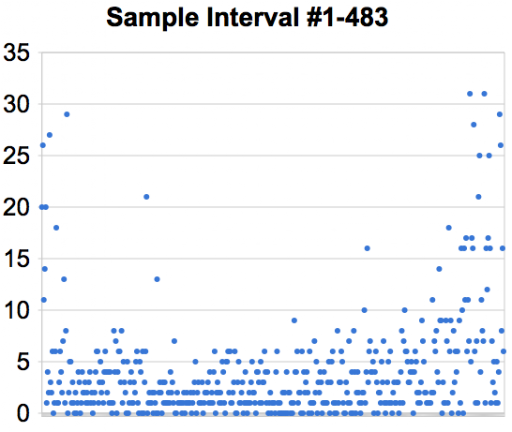# Nicholas Rinard Keene's Little Bit

I don't have a lot to say, but this is my little bit.

## Tuesday, September 29, 2009

### Genetic Algorithm Efficiency GraphThis is a graph summarizing 500 runs of the string-finding genetic algorithm from yesterday. In this graph, each dot represents the number of generations the algorithm required to find an 88-character string using 50 organisms with 3 offspring per organism per generation. Also, the chance of an organism experiencing a mutation was one in three, and a mutation could change one, two, or three characters in the organism's string.

For the most part there is a fairly linear distribution between 1,250 and 2,250 generations. Below 1,250, the frequency of occurance drops off slightly, never seeming to get below 900 (941 was the lowest of the 500 samples). Above 2,250 there is a sort of long tail where the algorithm took two or three times the average number of generations.The actual average was 1791 generations. If we ignored the tails below and above 1,250 and 2,250, the average is 1708. Let's look more closely at that long tail by considering the interval between each sample. The interval is the scalar distance between the previous sample and the current one, so if the current instance of the algorithm took X generations and the previous instance took Y generations, then the interval is X - Y. This tells us approximately the slope of the line formed by the samples, in between each sample.

The first of these interval graphs shows the intervals for samples 1 through 483. I chose that range because the greatest interval in that range is 31. Even in this range, you can see that the intervals greater than 10 occur primarily at the two ends.

Now let's look at the intervals in the tail, above sample 484, where you see the intervals range up to several hundred, far outside the previous range. In fact you can see that the very last sample required more than 500 additional generations to complete than its nearest sample. In fact within the tail, more than half (nine of seventeen) of the intervals are greater than any single interval in the other range.

This exposes a potential weakness of naive random genetic algorithms: sometimes the randomness just doesn't work out for you, requiring many additional generations. The worst sample in my set took took 2.5 times longer than the average, and nearly five times as long as the best sample. If I ran 50,000 instances instead of 500, I would probably get more pronounced tails on each end.